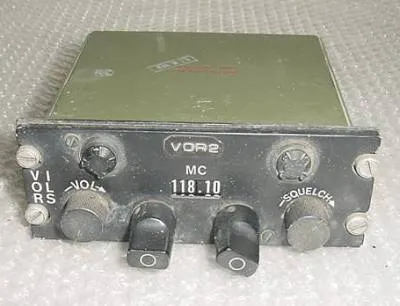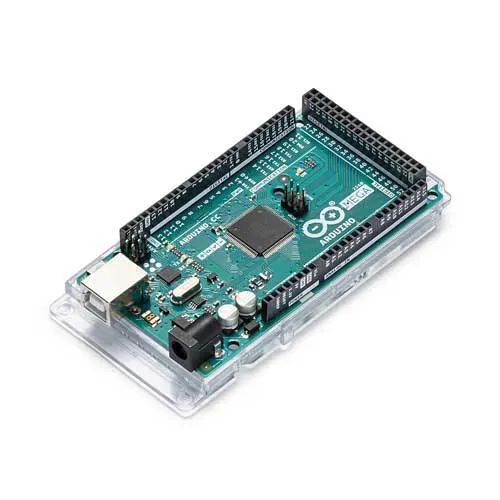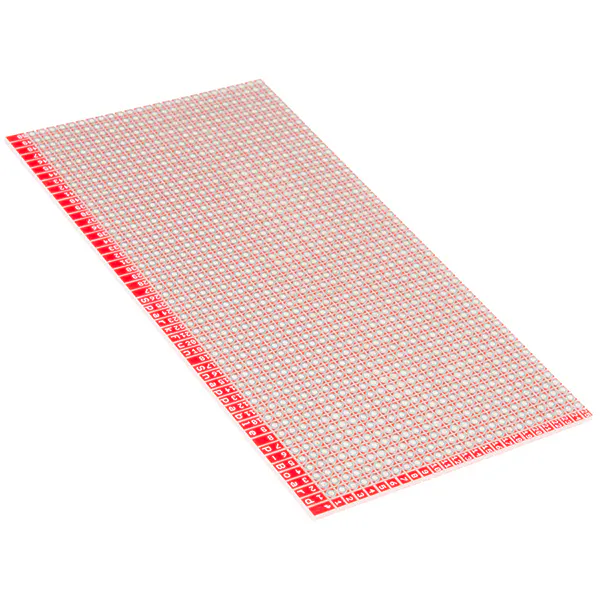Project showcase• 5,640 views
• 7 respects

## Components and suppliesArduino Mega 2560
×1
×1
 Relay (generic) This relay is Arduino ready
×1
 Nylon Screws & Nuts 4-40
×1
 Molex Right Angle Power Connector #15-24-4441
×1Jumper wires (generic)
×1
 Wire Wrap IC Socket -16 pin
×4
 Wire Wrap Female DB-25
×2SparkFun Snappable Protoboard
×1

## Apps and online services

The first rule is to do No Harm to the avionics, in this case, the radio head. The head is C-3436A/ARN-30. On the Internet, I found TM 11-1520-211-35 which is the devices manual. So it's has 3 ARC connectors on the back and we could not get the pin-out. Luckily I had built an interface cable a few years ago. The Cable has three(3) ARC connectors on one end and two (2) male DB-25 females on the other. So grab the DVM and start pinning it out.

So you have your pin out so now what?

Please Note: The ARC family of connectors where used from before WWII to the start of Vietnam, they are very hard to find.

THE ANALYSIS: OK, this radio is like most others but simpler, and not ARINC-410.  So I have to define what goes in (commands) and what comes out (information). The information side will be called Transmit and the command side Receive.

Transmit Side

• Power Switch: Two States: On - Off
• Frequency: 108 to 126 in unit steps, plus a Blank
• Frequency Fractions: .00 to .90 in unit steps.
• Volume Control: Analog output
• Squelch Control: Analog output

• Panel Lights: Two States: On - Off
• Dial Lights: Two States: On - Off

Since the lamps are 24 volts, we will need a relay to drive them. Now I am old school so I normally wire-wrap my one-offs. Please note the Molex connector, part number 15-24-4441  is a standard power connector, normally found on your hard-drive. I also used Nylon 4-40 hardware (no shorts).

Lastly, I wrote a small python script to receive the output and put it on my screen.

## Code

This Test Script is run in a Linux Terminal Session. You must first find the USB port number "/dev/ttyACM0" with a program called lsusb.
```import serial

ser = serial.Serial (
port 	= '/dev/ttyACM0',
baudrate 	= 115200,
parity	= serial.PARITY_NONE,
stopbits	= serial.STOPBITS_ONE,
bytesize	= serial.EIGHTBITS,
timeout	= 10
)

while True:

ser.close ()
```
##### C-3436A.outlineArduino
This is the code outline, I like to outline my code as its easier to understand later
```radio outine
C-3436A.h
1. define pins arrays and inilize them. Fpin[] & Hpin[] and power and panel light
2. define what the pins will be.
pinMode(F[n], PULLUP);
pinMode(Plight, OUTPUT);
3. define data arrays frac[] & hund[] and power and panel light.
4. define alt data arrays frac[]0 & hund[]0 and power and panel light.
5. define and inialize the two look up arrays flu[]={} and hlu[]={}
6. define 4 test variables: ftest, htest, ftest0, htest0.

-----
void() {
void init()
}
-----
init.c
void init()
does reads on the arrays F[] & H[] and variable Pwr
and assigns them to frac0[], hund0[] and variable Pwr0
the values for ftest0, htest0 are calulated.
-----
FORMATS:

send_message()
START BYTE  (0-7)   == FF
hund 4 bits (8-11)  == hlu(htest)
frac 4 bits (12-15) == flu(ftest)
switches:
power (16) = pwr
unused (17-24)

recv_message()
START BYTE (0-7) == FF
COMMANDS (0-15):
1. Send Status Message
2. Set Plamp ON
3. Set Plamp OFF

-----
void loop()
check for input: Serial.Read(ifavilable()){ decode(); }
calulate the values:    ftest, Htest;
if( ftest != ftest0 or htest != htest0 or pwr != pwr0 ) {
swap variables and arrays. frac0[] = frac[], hund0[] = hund[], pwr0 = pwr }
else { do nothing };
```
##### C3436A.hArduino
This is my header file. It's used is to separate the variables from the code base.
```/*
* C-3436A.h
*
*  C. Harrison	Aug 21, 2014	Creation
*
*/

// we need to list the digital input pins:

// Pin defs
// please note: arrays F[] & H[] are being indexed 1-5
int F[]= { 0, 22, 23, 24, 25, 26 };
int H[]= { 0, 30, 31, 32, 33, 34 };

int powerpin = 20;
int dial_light = 40;
// now we need the variables & arrays

int frac;
int hund;
int frac0;
int hund0;
int pwr, pwr0;
int ftest, ftest0;
int htest, htest0;
```
##### C-3436A.inoArduino
This is the program I wrote to "test" the software. This software does not have my protocols for how the Arduino will talk and listen to the Radio Sub System in NexGenin place.
```/*
* C-3436A
*
*  C. Harrison	Aug 30, 2014	Creation
*
*/

#include "C3436A.h"

void setup(){
void modes();
Serial.begin(19200);
Serial.println("C-3436A awake");
void init();
}

void loop(){
int i;
//  check for input:
while( Serial.available()) {
if( data == '!') {
if( cmd = 1 ) {
// panel light}
if( dat = 0 ) {
digitalWrite( dial_light, LOW); }
if( dat = 1 ) {
digitalWrite( dial_light, HIGH); }
}}

for( i= 1; i <  6; i++ ) {
//  calulate the values:    ftest, Htest;
int ftest, htest, ftest0, htest0, pwr, pwr0;

ftest = decode_F(calc_F());
htest = decode_H(calc_H());

if( ftest != ftest0 or htest != htest0 or pwr != pwr0 ) {
swap();  }
else {
; }  // Do Nothing
}}

int decode_H( int data ){
int code[] = {{13,0},{9,108},{11,109},{5,110},
{27,111},{16,112},{30,113},{2,114},
{23,115},{8,116},{28,117},{1,118},
{7,119},{4,120},{24,121},{20,122},
{6,123},{18,124},{17,125},{10,126}};

for( int i= 0; i < 22; i++ ) {
if( data == code[i]) {
return code[i]; }
}}

int decode_F( int data ){
int code[] = {20, 5, 8, 2, 16, 4, 1, 9, 10, 18 };

for( int i= 0; i < 10; i++ ) {
if( data == code[i]) {
return i; }
}}

int calc_F(){
int temp = ( F + (F*2) + (F*4) + (F*8) + (F*16));
return( temp );
}

int calc_H(){
int temp = ( H + (H*2) + (H*4) + (H*8) + (H*16));
return( temp );
}

void swap(){
for( int i = 1; i < 6; i++ ) {
frac0[i] = frac[i];
hund0[i] = hund[i]; }
ftest0   = ftest;
htest0   = htest;
pwr0     = pwr;
}

void modes(){

pinMode( F, INPUT_PULLUP);
pinMode( F, INPUT_PULLUP);
pinMode( F, INPUT_PULLUP);
pinMode( F, INPUT_PULLUP);
pinMode( F, INPUT_PULLUP);

// hundreds array
pinMode(H, INPUT_PULLUP);
pinMode(H, INPUT_PULLUP);
pinMode(H, INPUT_PULLUP);
pinMode(H, INPUT_PULLUP);
pinMode(H, INPUT_PULLUP);

// Now we have to assign the power switch pin

pinMode( powerpin, INPUT_PULLUP);

// Now we have to assign  the digital output pins

pinMode(dial_light, OUTPUT);

}

// we need to read the first time so while in loop the test will not fail.
// read all the pins and put then in arrays
void init(){
int i;

for( i=1; i< 7; i++){
ftest0   = decode_F(calc_F());
htest0   = decode_H(calc_H());
```

## Schematics

C-3436A.ods

February 6, 2016

#### Members who respect this project

See similar projects
you might like

#### Making Arduino-Based RC Transmitter of USB Flight Simulator

Project showcase by tsar_

• 8,988 views
• 12 respects

#### Arduino Altair 8800 Simulator

Project tutorial by David Hansel

• 100,407 views
• 193 respects

#### Arduino Traffic Light Simulator

Project tutorial by Zachary Haslam

• 77,566 views
• 100 respects

#### DIY Flight Instruments for Horizon and Compass

Project tutorial by Aritro Mukherjee

• 52,611 views
• 93 respects

#### Simple Programmable Robotic Arm

Project showcase by Ryan Chan

• 165,431 views